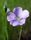## Anything wrong with the code?

Hello,

I tried all I can to check the following code, but still cannot figure out why I cannot get what I want for the first column, should be, Total Amt, Score, Regained %, instead of numbers. and the % of Variance column should be the % format, instead of numbers,  Please see that attachment for the graph that is supposed to be.  Thank you for your help!

FILENAME mail

email to="famizhang@gmail.com"

cc=("famizhang@gmail.com")

SUBJECT="Report" CONTENT_TYPE="text/html";

ods listing close;

stylesheet=(url="temp.css") options(pagebreak='no')    ;

title1 j=c COLOR=white " "    ;

proc format ;

picture million (round default=32)

-1E15 -< -1E12 = '009.9T' (prefix='-\$' mult=1E-11)

-1E12 -< -1E09 = '009.9B' (prefix='-\$' mult=1E-8)

-1E09 -< -1E06 = '009.9M' (prefix='-\$' mult=1E-5)

-1E06 -< -1E03 = '009.9K' (prefix='-\$' mult=1E-2)

1E03 -<  1E06 = '009.9K' (prefix='\$' mult=1e-2)

1E06 -<  1E09 = '009.9M' (prefix='\$' mult=1e-5)

1E09 -<  1E12 = '009.9B' (prefix='\$' mult=1E-8)

1E12 -<  1E15 = '009.9T' (prefix='\$' mult=1E-11);

run;

PROC FORMAT;

VALUE negfmt

low-<0 = 'red'

OTHER = 'black';

RUN;

Proc report data=allproduct  contents="   " nowd split='\'

style(column)={color=white just=center background=lightcyan foreground=black };

column _name_   month2016 month2015 Num_of_Variance Perc_of_Variance   year2016 year2015  Perc_of_Variance_y;

define _name_ / "Dollars"   style=[fontstyle=Roman  fontweight=bold width=2in font_size=2.2 cellwidth=2in just=center foreground=black];

define month2016/  "mon16" style=[fontstyle=Roman  fontweight=bold width=0.7in font_size=2.2 cellwidth=0.7in just=center foreground=negfmt.];

define month2015/  "Mon15" style=[fontstyle=Roman  fontweight=bold width=0.7in font_size=2.2 cellwidth=0.7in just=center foreground=negfmt.];

define Num_of_Variance/  "# of Variance"  style=[fontstyle=Roman  fontweight=bold width=0.7in font_size=2.2 cellwidth=0.7in just=center foreground=negfmt.];

define Perc_of_Variance/  "% of Variance"   style=[fontstyle=Roman  fontweight=bold width=0.7in font_size=2.2 cellwidth=0.7in just=center foreground=negfmt.];

define year2016/  "16YTD"  style=[fontstyle=Roman  fontweight=bold width=0.7in font_size=2.2 cellwidth=0.7in just=center foreground=negfmt.];

define year2015/  "15YTD" style=[fontstyle=Roman  fontweight=bold width=0.7in font_size=2.2 cellwidth=0.7in just=center foreground=negfmt.];

define Perc_of_Variance_y/  "% of Variance "   style=[fontstyle=Roman  fontweight=bold width=0.7in font_size=2.2 cellwidth=0.7in just=center foreground=negfmt.];

%macro comp(arg);

compute &arg;

if _name_='Total Amt'   then call define(_col_,'format','million.');

if _name_='Score’ then call define(_col_,'format','comma8.0');

if _name_= 'regained %' then call define(_col_,'format','percent8.1');

endcomp;

%mend common_lines;

%comp(_name_);

%comp(month2016);

%comp( month2015);

%comp( Num_of_Variance);

%comp(Perc_of_Variance);

%comp(year2016);

%comp(year2015);

%comp(Perc_of_Variance_y);

RUN;

1 ACCEPTED SOLUTION

Accepted Solutions

## Re: Anything wrong with the code?

You show what you want but now what you are getting. Also do you get any notes in the log?

I suspect the issue has to do with

if _name_= 'regained %' then call define(_col_,'format','percent8.1');

possibly either existence, spelling or case of 'regained %'.

2 REPLIES 2LinusH
Tourmaline | Level 20

## Re: Anything wrong with the code?

Try to narrow down your problem. Even if it seems simple to you, it's not always to trouble shoot "on paper".

I would start to leave out the macro.

If you still can't figure it out, you need to attach some test data in the form of datalines/cards.

Also, have the macro defined outside (prior) the PROC REPORT step.

Data never sleeps

## Re: Anything wrong with the code?

You show what you want but now what you are getting. Also do you get any notes in the log?

I suspect the issue has to do with

if _name_= 'regained %' then call define(_col_,'format','percent8.1');

possibly either existence, spelling or case of 'regained %'.

Discussion stats
• 2 replies
• 870 views
• 0 likes
• 3 in conversation